+关注继续查看

# 二、计算几率

• 概率是非负的：对于每个事件A• 整个空间的概率为 1：## 加法

def show_disjoint_union():
plt.figure(figsize=(10, 20))
# create the circles with shapely
a = sg.Point(1.4,2.5).buffer(1.0)
b = sg.Point(3.3,2.5).buffer(0.75)

# use descartes to create the matplotlib patches
ax = plt.subplot(121)
ax.annotate('A', [1.4, 2.5])
ax.annotate('B', [3.3, 2.5])

# control display
plt.title('Mutually Exclusive Events')
plt.axis('off')
ax.set_xlim(0, 5); ax.set_ylim(0, 5)
ax.set_aspect('equal')

# use descartes to create the matplotlib patches
ax = plt.subplot(122)

# control display
plt.title('Disjoint Union')
plt.axis('off')
ax.set_xlim(0, 5); ax.set_ylim(0, 5)
ax.set_aspect('equal')

show_disjoint_union()### 第三个公理，加法规则

• 如果AB是互斥事件，那么• 对于任何固定的n，如果是互斥的（也就是对于所有），那么：有时叫做有限可加性公理。

### 嵌套事件def show_difference():
plt.figure(figsize=(10, 20))
# create the circles with shapely
a = sg.Point(2,2.5).buffer(1.0)
b = sg.Point(2,2.5).buffer(0.75)

# compute the 2 parts
left = a.difference(b)
middle = a.intersection(b)

# use descartes to create the matplotlib patches
ax = plt.subplot(121)

# control display
plt.title('Nested Events')
plt.axis('off')
ax.set_xlim(0, 5); ax.set_ylim(0, 5)
ax.set_aspect('equal')

# use descartes to create the matplotlib patches
ax = plt.subplot(122)

# control display
plt.title('The Difference')
plt.axis('off')
ax.set_xlim(0, 5); ax.set_ylim(0, 5)
ax.set_aspect('equal')

show_difference()### 减法规则### 补集

#### 补集规则

def show_complement():
plt.figure(figsize=(10, 20))
# create the square and circle with shapely
a = sg.box(0, 0, 4.5, 4.5)
b = sg.Point(2.25,2.5).buffer(1)

# compute the 2 parts
left = a.difference(b)
middle = a.intersection(b)

# use descartes to create the matplotlib patches
ax = plt.subplot(121)

# control display
plt.title('An Event (Square = Omega)')
plt.axis('off')
ax.set_xlim(0, 5); ax.set_ylim(0, 5)
ax.set_aspect('equal')

# use descartes to create the matplotlib patches
ax = plt.subplot(122)

# control display
plt.title('The Complement')
plt.axis('off')
ax.set_xlim(0, 5); ax.set_ylim(0, 5)
ax.set_aspect('equal')

show_complement()## 示例

A成为事件“我们得到至少一个正面和至少一个反面”。问题要求P(A)。因为是事件“序列的所有元素都相同”，所以我们有：## 示例 2：骰子的 12 次投掷的最大值12 次投掷没有什么特别之处。你可以在整个过程中用n代替 12，并且参数将如上所述。

### 示例 3：大于第一个随机数的第二个随机数

• 第一位数字 0，第二位 1 到 9
• 第一位数字 1，第二位 2 到 9
• 第一位数字 2，第二位 3 到 9
• 等等，直到
• 第一位数字 8，第二位 9## 乘法

def show_intersection():
plt.figure(figsize=(8, 16))
# create the circles with shapely
a = sg.Point(-.5,0).buffer(1.0)
b = sg.Point(0.5,0).buffer(0.75)

# compute the 3 parts
left = a.difference(b)
right = b.difference(a)
middle = a.intersection(b)

# use descartes to create the matplotlib patches
ax = plt.subplot(121)
ax.annotate('A', [-0.5, 0])
ax.annotate('B', [0.5, 0])

# control display
plt.title('Two Events')
plt.axis('off')
ax.set_xlim(-2, 2); ax.set_ylim(-2, 2)
ax.set_aspect('equal')

# use descartes to create the matplotlib patches
ax = plt.subplot(122)

# control display
plt.title('The Intersection')
plt.axis('off')
ax.set_xlim(-2, 2); ax.set_ylim(-2, 2)
ax.set_aspect('equal')

show_intersection()AB为两个事件。 交集A ∩ BAB都是发生的事件，右侧的维恩图中以亮蓝色显示。

### 无放回随机抽取### 条件概率AB为两个事件。 那么他们都发生的几率是：### 示例 1：两张牌中的两个 A### 示例 2：99 岁## 更多示例

### 示例 1：基本方法 - 从划分开始

（啊！这两项是相同的！为无放回抽样中的更多这样的对称性做好准备。）

### 示例 2：波利亚坛子模型P(second ball blackfirst ball black)=b+db+w+dP(second ball black∣first ball black)=b+db+w+d## 更新概率

### 示例：真阳性P(D+)=P(D and +)P(+)=0.0040.990.0040.99+0.9960.005=44.3%P(D∣+)=P(D and +)P(+)=0.004⋅0.990.004⋅0.99+0.996⋅0.005=44.3%

(.004*.99)/(0.004*.99 + 0.996*.005)
# 0.44295302013422816

### 贝叶斯规则### 先验的影响

• 我们将把疾病的“先验概率”从 0.004 改为其他值；“无疾病”的先验概率将相应做出改变。
• 我们将保持测试准确率不变。
• 我们将观察对于先验的不同值，给定某人是阳性，疾病的“后验概率”的变化。
prior = make_array(0.004, 0.01, 0.05, 0.1, 0.5)
Table().with_columns(
'Prior P(D)', prior,
'Posterior P(D|+)', (prior*0.99)/(prior*0.99 + (1-prior)*0.005)
)

0.004 0.442953
0.01 0.666667
0.05 0.912442
0.1 0.956522
0.5 0.994975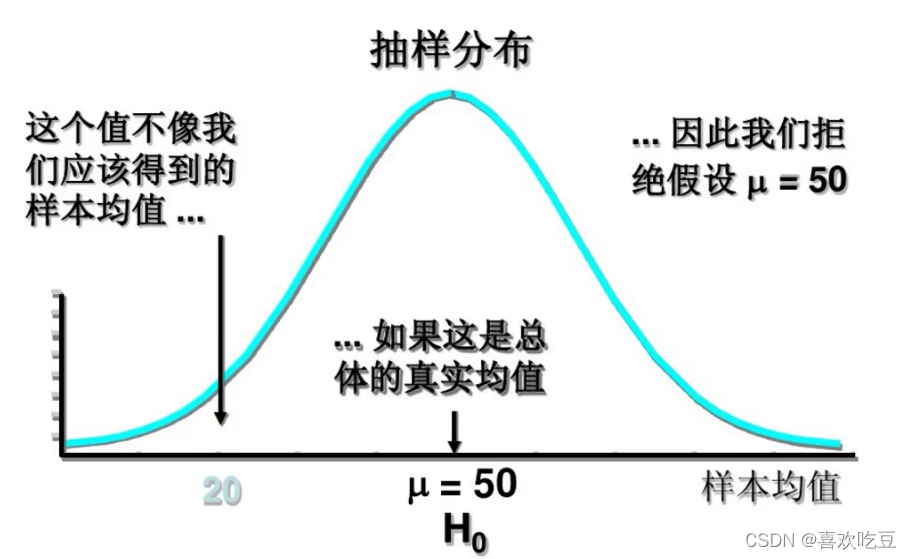27 0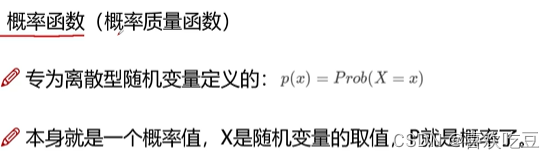25 0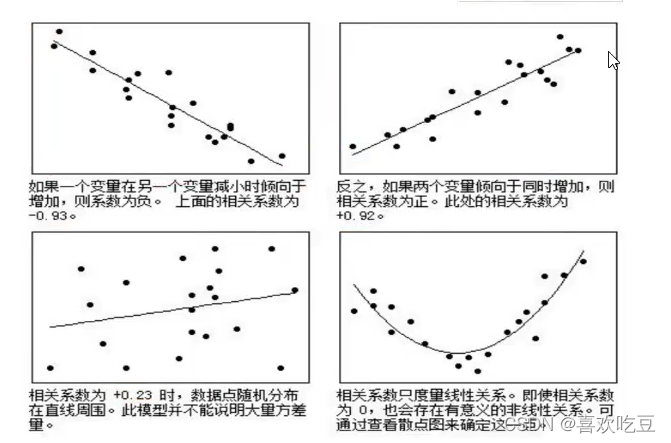r的绝对值表示变量之间的密切程度(即强度)。绝对值越接近1，表示两个变量之间关系越密切;越接近0，表示两个变量之间关系越不密切
16 0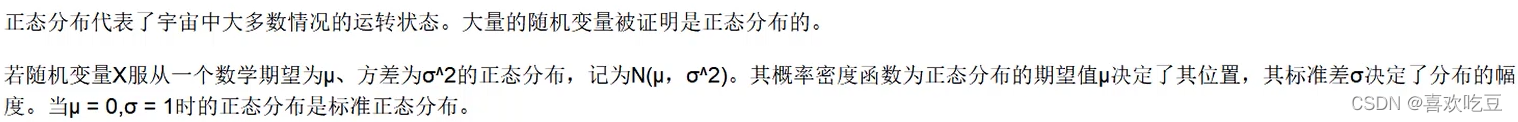11 0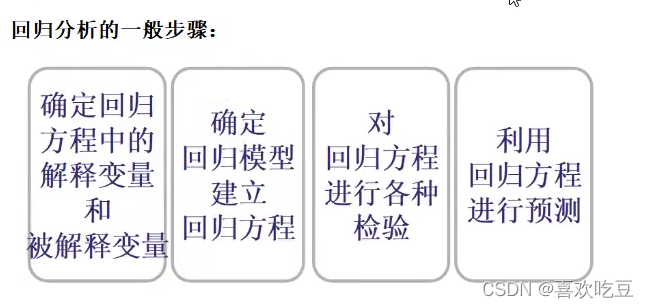12 0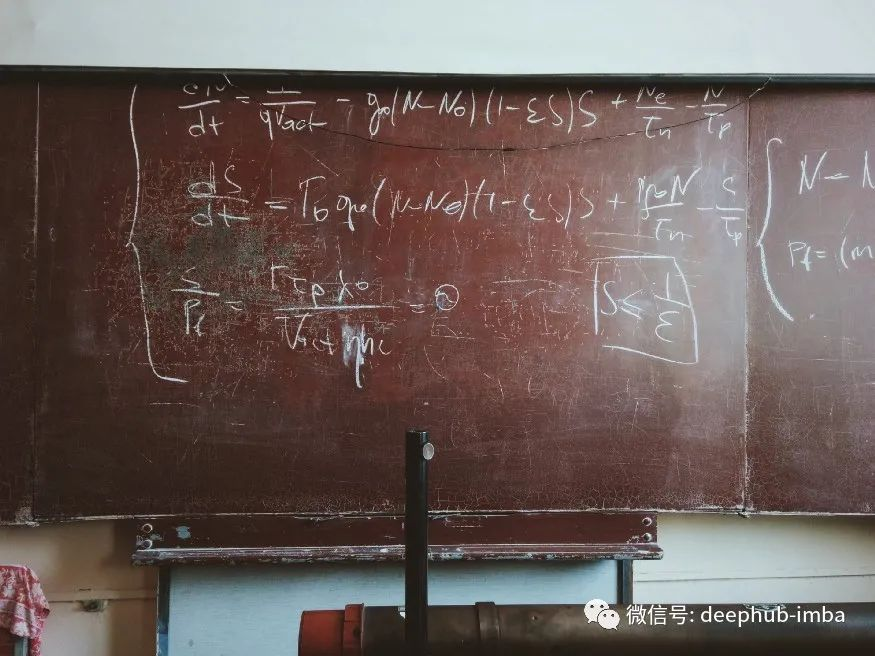19 0600 0
apachecn_飞龙
Github：@wizardforcel 简书：@ApacheCN_飞龙 微博：@龙雀 CSDN：@wizardforcel ApacheCN 官网：apachecn.org 机器学习交流群：62947023372413101059617

【算法实战】5. Logistic回归算法396

【算法实战】12. 利用 Apriori 算法进行关联分析562

【算法实战】9. 线性回归算法295